# Catch up on the latest AI articles# The Return Of ACGAN - ReACGAN

3 main points
✔️ Discovered that the source of instability in ACGAN training is the gradient explosion of discriminators
✔️ Proposed a new loss function D2D-CE that can also consider relationships between data
✔️ ReACGAN, an improved version of ACGAN, is developed to achieve image generation performance comparable to BigGAN.

written by Minguk KangWoohyeon ShimMinsu ChoJaesik Park
(Submitted on 1 Nov 2021)

Subjects:  Computer Vision and Pattern Recognition (cs.CV); Artificial Intelligence (cs.AI); Machine Learning (cs.LG)code：The images used in this article are from the paper, the introductory slides, or were created based on them.

## first of all

Previous research on adversarial generative networks (GANs) has been directed toward addressing problems such as mode collapse that impairs the diversity of the generated samples and the difficulty of training. Specifically, the definition of an objective function that does not cause gradient vanishing, the development of regularization methods that stabilize training, and the effective use of label data in data sets are some of the examples.

GANs that use label data in the dataset is specifically called Conditional GANs, and are classifier-based GANs (classifier-based GANs) or projection-based GANs ( projection-based GAN).

In discriminant-based GAN, the discriminator predicts not only whether the data is real or not but also its class label at the same time. The problems of the discriminator-based GAN are well known, such as mode collapse in the early stage of training when the number of classes increases, and the lack of diversity of the generated samples within a class in the image generation.

On the other hand, projection-based GAN adopts an architecture in which the inner product of the condition vector projected from the class labels and the feature vector of the data is computed in the discriminator. Since the only one-to-one relationship between data and labels is considered, there is still room to consider the relationship between data in a dataset.

In the paper presented in this paper, we develop an improved version of ACGAN, ReACGAN, which suppresses the gradient explosion problem by projecting the input onto a hypersphere and proposes a new loss function Data-to-Data Cross-Entropy (D2D-CE) that considers the relationship between data.

## Instability in ACGAN learning

In conventional GAN, the neural network that distinguishes between real and generated data is called Discriminator (D), whereas, in ACGAN, this discriminator also classifies the class labels of objects in the image.

Cross-entropy is used as the loss function for this class classification, and when this loss function is calculated in a neural network with a softmax layer, the partial derivative concerning the weights of the final linear layer is calculated as the following equation.The $1_{y_i}=k$ represents a function that takes 1 if the class label $y$ is $k$ and 0 otherwise, and $p_{i,k}$ represents the probability that sample $i$ belongs to class $k$.

We found that the small value of $p_{i,k}$ in the early stage of training causes the gradient norm to increase, which leads to mode collapse and makes the ACGAN learning unstable.

In the original paper, it is confirmed that simply normalizing the output $F(x)$ of the final intermediate layer in the discriminator (setting the norm of the above equation to 1) is enough to obtain the effect of learning stabilization.

## Data-to-Data Cross-Entropy Loss (D2D-CE)

As mentioned above, in projection-based GAN, only the relationship between data and class labels can be considered, while ReACGAN proposes a loss function D2D-CE that can also consider the relationship between data.

In D2D-CE, given that the conventional cross-entropy is calculated using the inner product between the feature vector corresponding to the class label (the weight vector in the final linear layer) and the feature vector extracted from the data, this inner product is calculated even between samples belonging to different classes, so that the data can be relationship is reflected.

Specifically, D2D-CE can be expressed by the following equation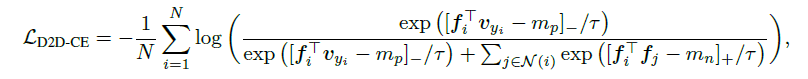In the equation $f$ is the normalized embedding representation obtained by inputting the image into the feature extractor ($F$) and further passing it through the projection layer, $v$ is the normalized embedding representation corresponding to the class and $\tau$ is the temperature parameter. The $N(i)$ represents the set of samples belonging to different classes. Here normalization refers to the normalization to set the gradient norm to 1 described in the previous section.

What kind of effect do you expect by introducing a loss function that takes into account the relationship between the data in this way? The figure below shows how the learning progresses as the samples are placed in the feature space in the training of each model. In the figure, blue represents the non-canine class, red represents the canine class, ★ represents the linear layer weight $w$ of the classifier, and the arrow represents the direction of learning.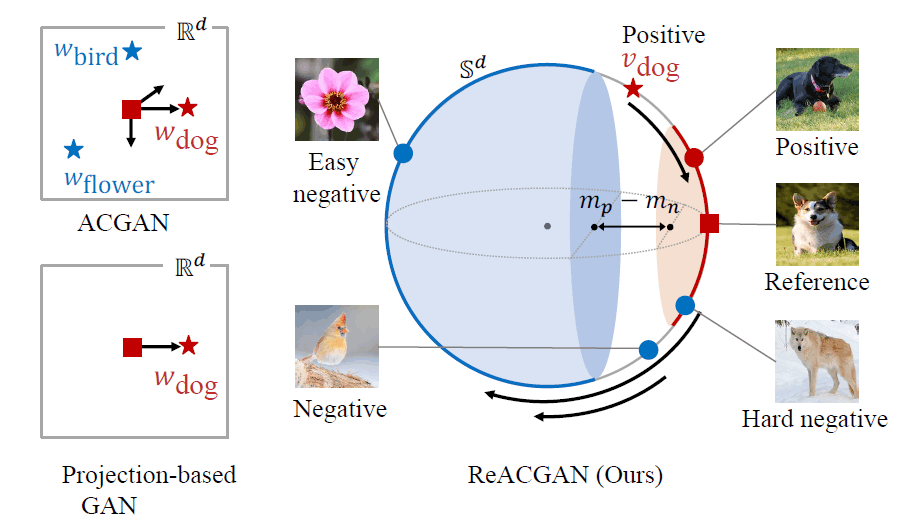In discriminator-based GAN, the weights are updated so that the samples move away from different classes and closer to the class to which they belong. In projection-based GAN, the weights are updated so that the samples are closer to the class to which they belong.

On the other hand, in the training of ReACGAN using D2D-CE, the introduction of a margin term ignores samples that can be easily classified into different classes, and the weights are updated so that negative examples, which are difficult to discriminate, are kept away from positive examples and closer to the class they belong to. This is expected to ensure the variability of the samples within a class while maintaining the separability between classes.

Experimental results show that D2D-CE also contributes to training stability: the feature map norm and the gradient norm of the classifier were calculated for each iteration on the Tiny-ImageNet dataset, and each value remained low after the introduction of D2D-CE. It is suggested that D2D-CE suppresses gradient explosion and contributes to training stability.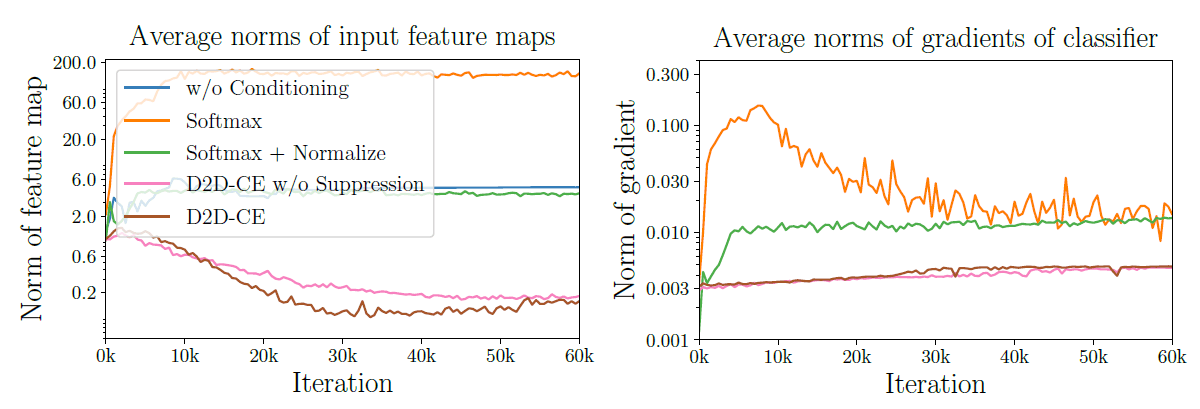## Overall view of ReACGAN

The overall picture of ReACGAN is shown in the figure below. the major change from ACGAN is that a new loss function is introduced to judge whether the samples belong to the same class or not, rather than classifying them.

D2D-CE is unique in that it computes the inner product of feature vectors between samples belonging to different classes, so a symmetric matrix (false negatives mask) is used to indicate whether the classes are the same or not between the samples.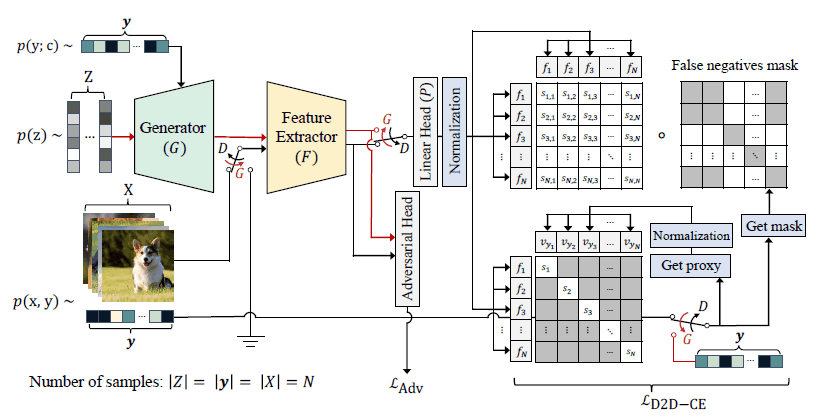## ReACGAN Performance

The images generated by ReACGAN are shown below for five benchmark datasets.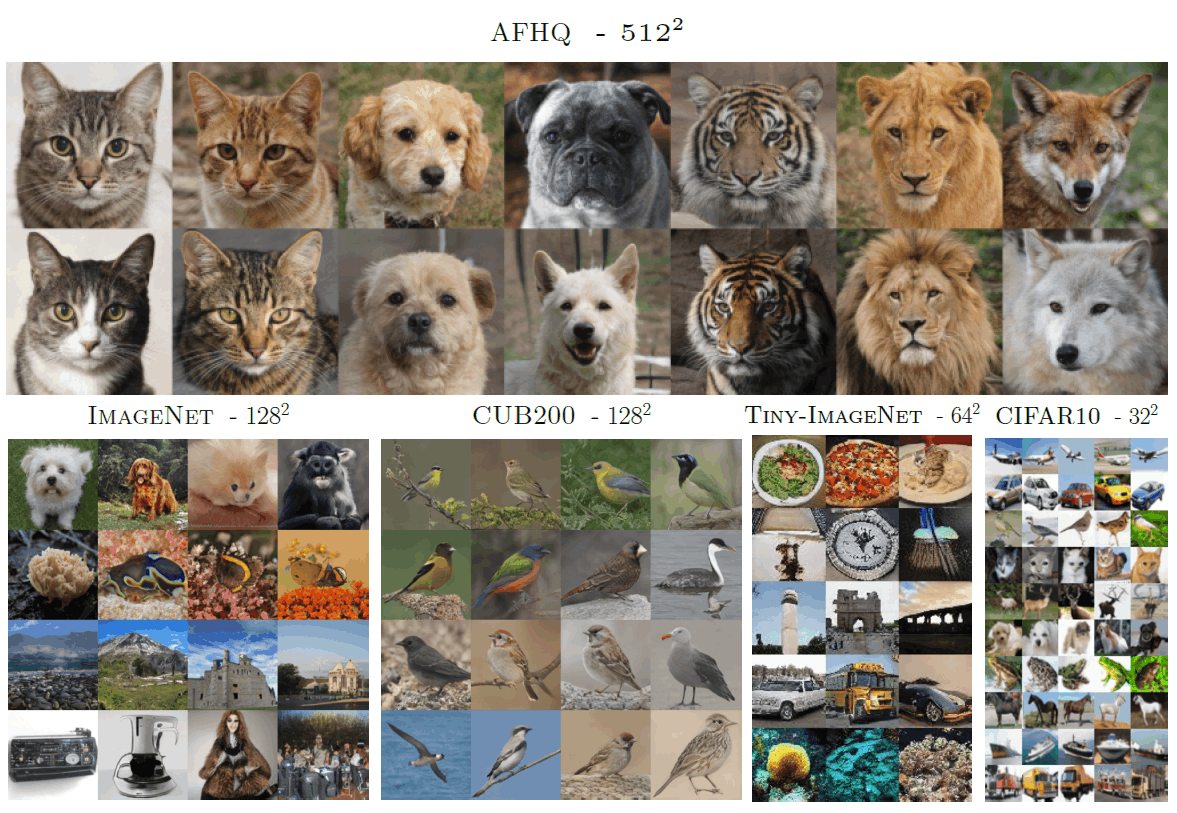The generation capability is much improved compared to the previous ACGAN. However, it should be noted that the size of the network parameters has increased significantly with the BigGAN architecture.

The results of evaluation by Inception Score(IS) and Frechet Inception Distance(FID) in ImageNet are as follows.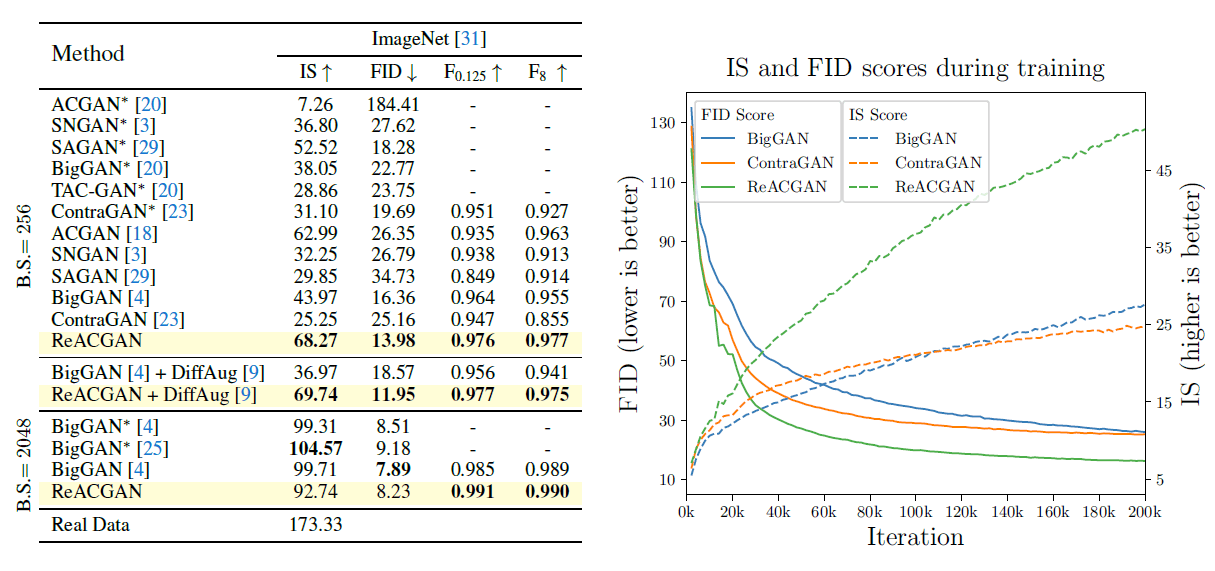ReACGAN has a high generation capability when the batch size is relatively small (256) and loses to BigGAN when the batch size is as large as 2048.

The learning curve shows that IS tends to rise faster and FID tends to fall faster in ReACGAN. This may be due to the effect of training stabilization in the early stage of learning.

You may consider training with ReACGAN if you cannot increase the batch size anyway to increase the reliability of the gradient, such as in an environment with low memory.

## summary

I titled it as the second coming of ACGAN, but I felt that it is closer to ContraGAN than ACGAN in terms of the design of loss function and so on.

The results of comparing the proposed loss function D2D-CE with other loss functions and applying it to different architectures can be found in the original paper, so please refer to it if you are interested.

If you have any suggestions for improvement of the content of the article,